## r plot program example and how to use it.

R Plot Function is used to plot a graph in r programming in this r function we can vector and then we get a graph which is about  magnitude vs index.

r plot function has so many method which is called according to the passed value to this r plot function.

For example we pass two vector plot(c(1,2),c(3,5)) and then this function plot a scatter graph for these two point.

here we plot graph for sine function form range -pi to pi as you can see in this picture.

```<- pi="" plot="" pre="" seq="" sin="" x="">```x <- seq(-pi,pi,0.1)
plot(x, sin(x))``````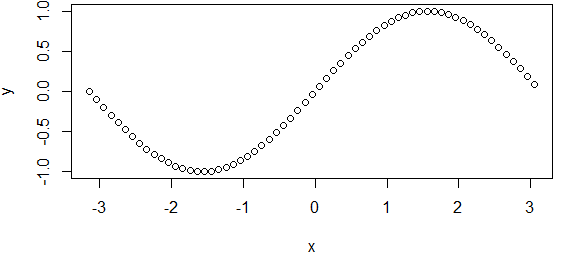R Plot Function

# Adding Titles and Labeling Axes to the r plot function

Here you can add title and label to the with parameter main and xlab and ylab two parameter for labeling x-axis and y-axis in plot graph.

``````plot(x, sin(x),
main="The Sine Function",
ylab="sin(x)")``````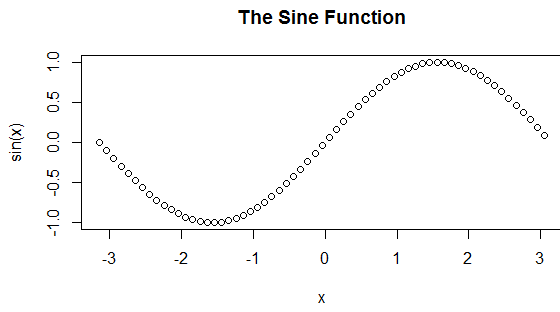Labeling Axes to the r plot function

# Changing Color and Plot Type for r function : -

Well you can change the color for plot type with the help of the argument type in r languages.

Check out the example for r plot with color:-

``````plot(x, sin(x),
main="The Sine Function",
ylab="sin(x)",
type="l",
col="blue")``````

here we can define the color with col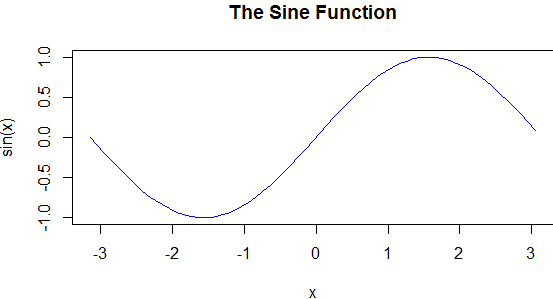changing Color and Plot Type

## Use of clrscr function in c with example

clrscr function in C programming is use to clear the screen and move the arrow upper left-hand corner of your computer screen.

this function work only for the GCC compiler otherwise use clear/cls command.

# Example program for clrscr function in c

```#include<stdio.h>
#include<conio.h>

int main()
{
printf("Press any key to clear the screen.\n");

getch();

clrscr();

printf("This appears after clearing the screen.\n");
printf("Press any key to exit...\n");

getch();
return 0;
}```

As you can see with the help of printf function computer is asking to press any key to clear the screen and then the second message will be displayed.

## Example program for pow function in c

pow function c programming is use to computer the power of a given number to the function.

pow() c function takes two arguments first one is known as base and second one as power value and pow() c function return a base with the power raised to that base number.

## Syntax for pow() c

`[Mathematics] xy = pow(x, y) [In programming]`

pow c function defines under the math.h header file in c.

If you want to find the pow of any int and float number then convert the operator type into the double.

```int base = 3;
int power = 5;
pow(double(base), double(power));```

# Example for C pow() function

```#include <stdio.h>
#include <math.h>```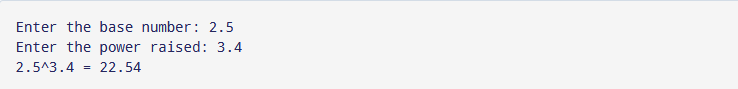program output for pow function in c

## Write a program for feof function in c

feof function in c programming is used for file handling.

feof c use to find the end of an file.

## Syntax for feof c program :-

`int feof(FILE *fp)`

# Example program for feof function in c

```# include <stdio.h>
int main( )
{
FILE *fp ;
char c ;
printf( "Opening the file test.c in read mode" ) ;
fp = fopen ( "test.c", "r" ) ; // opening an existing file
if ( fp == NULL )
{
printf ( "Could not open file test.c" ) ;
return 1;
}
printf( "Reading the file test.c" ) ;
while ( 1 )
{
c = fgetc ( fp ) ; // reading the file
if( feof(fp) )
break ;
printf ( "%c", c ) ;
}
printf("Closing the file test.c as end of file is reached.");
fclose ( fp ) ; // Closing the file
return 0;
}```

This file handling program use to read a open file and feof c function used to find the end of the file.program output for feof c

## Example for rand function in c programming

rand function in c programming is used to generate the pseudo-random numbers with the help of rand c function.

rand c function return the pseudo-random numbers which is exist between 0 and RAND_MAX.

rand function in c didn't use any parameters.

Syntax for rand c : -

`int rand(void)`

# Example program for rand c

```#include <stdio.h>
#include <stdlib.h>

int main () {
int i, n;
time_t t;

n = 5;

/* Intializes random number generator */
srand((unsigned) time(&t));

/* Print 5 random numbers from 0 to 49 */
for( i = 0 ; i < n ; i++ ) {
printf("%d\n", rand() % 50);
}

return(0);
}```output program for rand c

## Example program for strcat() function in c

If you have a computer programming background then we believe you heard the name of concatenation this word in programming language is known for joins to strings.Example program for strcat() function in c

Here in c language strcat() function in c use to joins two given string in c programs.

## Syntax for strcat c

`char *strcat(char *destination, const char *source)`

strcat c function defines in string.h header file.

## strcat() c arguments

strcat() function use two arguments

• destination - first one is destination string.
• source - source of the string is second one.

strcat() function in c add  destination string and the source string and final result will be stored in destination string.

# Example program for strcat() c

```#include <stdio.h>
#include <string.h>
int main() {
char str1 = "This is ", str2[] = "functioninc.in";

// concatenates str1 and str2
// the resultant string is stored in str1.
strcat(str1, str2);

puts(str1);
puts(str2);

return 0;
}```

## Example program for sprintf c function

•  sprintf c function in used to convert any integer into a string.
•   sprintf function in c didn't send the output to the console return an formatted string.

# Syntax for sprintf c function

`int sprintf(char *str, const char *control_string, [ arg_1, arg_2, ... ]);`
•  First argument is pointer to a targeted string for sprintf function in c.
•  Other arguments are same like printf() function in c.
• sprintf function in c use a variable str to write the data and give the number characters written to str.
• sprintf c return the -1 if any error occurs.

# Example for sprintf c function : -

```#include<stdio.h>
#include<string.h>
int factorial(int );

int main()
{

int sal;
char name, designation, info;

printf("Enter your name: ");
gets(name);

printf("Enter your designation: ");
gets(designation);

printf("Enter your salary: ");
scanf("%d", &sal);

sprintf(info, "Welcome %s !\nName: %s \nDesignation: %s\nSalary: %d",
name, name, designation, sal);

printf("\n%s", info);

// signal to operating system program ran fine
return 0;
}```program output for sprintf function in c

## fopen function in c programming with it's example

fopen c function is used to open a file which is denoted by the file name in c programming.

Syntax for fopen function in c : -

`FILE *fopen(const char *filename, const char *mode)`

## Parameters For for fopen c : -

filename : - this element contain the file name which is going to be open.

Mode :- This string in c contain the method to access the file.

Check the mode for open a file in c programming with the help of fopen c function.

• "r" Mode : - This mode opens a file for reading.
• "w" Mode : - this one use to create a empty file for writing.
• "a" Mode : - It use to append the data  at the end of the file.
• "r+" Mode : - This mode open a file to make an update in reading and writing.
•  "w+" Mode :  - Create an empty file for both reading and writing.
•  "a+" Mode : - Use to open an file for appending and also for reading.

Return Value for fopen c function : -

•  fopen function in c return a file pointer otherwise give a null.
• errno global variable is used to indicate the error in fopen function.

# Example program for fopen function in c :

```#include <stdio.h>
#include <stdlib.h>

int main () {
FILE * fp;

fp = fopen ("file.txt", "w+");
fprintf(fp, "%s %s %s %d", "We", "are", "in", 2012);

fclose(fp);

return(0);
}```Example program for fopen c

## Example program for fread function in c

fread function in c used to read the data from desired file and stored it into a buffer.

syntax For fread c: -

`size_t fread(void * buffer, size_t size, size_t count, FILE * stream)`

## Parameters For fread in c

fread c use 4 parameters in it's program and these parameters are follow  :-

Buffer : -  buffer is also know as the space which is used to temporarily stored the data.

it did the same work for fread function in c.

Size: This one is an another parameters which is used to read the size in byte for each elements.

Count: Used to count the number of elements (like file for fread in c).

Stream :- Pointer is used for file object to tell the place where data is going to be read.

# Example for the fread c

```#include <stdio.h>

int main() {
char buffer; // Buffer to store data
FILE * stream;
stream = fopen("file.txt", "r");
int count = fread(&buffer, sizeof(char), 20, stream);
fclose(stream);
// Printing data to check validity
printf("Data read from file: %s \n", buffer);
printf("Elements read: %d", count);
return 0;
}```
OUTPUTfread c

## Typecast Functions Like atoi in c,atof, itoa, atol more

atoi in c is used to convert the string data type into int data type.atoi function in c

Syntax for atoi in c:-

`int atoi (const char * str);`

atoi in c function in c is used with the “stdlib.h” header file Well this function is also known as the typecast functions in C.

# Example program for the atoi in c

`#include <stdio.h>`
```#include <stdlib.h>

int main()
{
char a = "100";
int value = atoi(a);
printf("Value = %d\n", value);
return 0;
}```

Lets check the similar typecast functions Like atoi in c.

atof() function in c  :- atof function work similar like atoi the only difference is atof in c used to converts string data type into float data type.

# Example program for atof() function in c :-

```#include <stdio.h>
#include <stdlib.h>

int main()
{
char a = "3.18";
float pi = atof(a);
printf("Value of pi = %f\n", pi);
return 0;
}```

atol() function in c  :-  This function is used to change the string data type to long data type like it's name(atol).

Syntax for atol in c:  -

`long int atol ( const char * str );`

# Example program for atol() function in c :-

```#include <stdio.h>
#include <stdlib.h>

int main()
{
char a = "100000000000";
long value = atol(a);
printf("Value = %ld\n", value);
return 0;
}```

itoa() function in c  :- This function is reversed of atoi in c, itoa function is used to convert the int data type to string data type.

Syntax : -

`char *  itoa ( int value, char * str, int base );`

# Example program for itoa() function in c

```#include <stdio.h>
#include <stdlib.h>
#include <string.h>

int main()
{
int a=54325;
char buffer;
itoa(a,buffer,2);   // 2 means decimal
printf("Binary value = %s\n", buffer);

itoa(a,buffer,10);   // 10 means decimal
printf("Decimal value = %s\n", buffer);

itoa(a,buffer,16);   // 16 means Hexadecimal
printf("Hexadecimal value = %s\n", buffer);
return 0;
}```

## Example Program for memset function in c

memset() function in C use to take a memory location and taken a VOID* pointer and this function copies the first n byte in memory location.

After update the memory location it return that location with the help of pointer.memset c

## Syntax :  -

`void* memset(void* mem_loc, int c, size_t n);`

mem_loc is a memory location

c is an unsigned character in that upper syntax

# Header File for memset function in c

Here this function deal with the characters so we have to use header file in the program

Now the complete import for memset function

````#include <string.h>`

void* memset(void* mem_loc, int c, size_t n);```

# Program Example for memset c

`#include <stdio.h>`
`#include <string.h>`
```
int main() {
char a[] = {"Hello from functioninc"};

printf("a = %s\n", a);

printf("Filling the first 5 characters a with 'P' using memset\n");

memset(a, 'P', 5 * sizeof(char));

printf("After memset, a = %s\n", a);

return 0;
}```

Then the 5 character of the given string is filled by the 'P'

```a = Hello from Functioninc
Filling the first 5 characters with 'P' using memset function in c
After memset c, a = PPPPP from Functioninc```

# Conclusion of memset c

Here we learn about the memset function in c and how to allocate the memory location.

## Understand the extern in c with extern c example

•  extern keyword in C is used when we have multiple source file and we want to shear the variable among those files.
• Or when we prefixing a global variable with the extern keyword in C that's mean we are telling to the compiler that the variable is already defined in other file.
• That's means don't allocate any memory for the same variable twice.

• ## A variable definition statement

declaration statement for extern

```int a;

char* ch;```

With the help of extern in c we can tell to the compiler that these variables already exist somewhere so don't allocate any memory for these variables.

There is an another way to defining a variable,where you can allocate storage for the given variable.

```// Defining a
int a = 11;

// Defining ch, even if it is NULL
char* ch = NULL;```

let's check the use of extern in c. You can use this extern keyword only for declaring a global variable.

```// Allowed. Variable declaration
extern int a;

// Not allowed. extern with variable definition
extern int b = 5;```

# Let's know about the use of extern in c with example

Assume we have to files

•     file1x.c, file1x.h
•     file2x.c file2x.h.

And a main.c which is an drive program.

Here we have a global variable which is called int file1_var defined in file1x.c.

Here we use extern in extern c example for sharing the same variable for file2x.c.

Here the extern c example : -

```// file1.h
// Contains function prototypes for functions of file1.c

int addition_1(int a, int b);```

```// file1.c
// Contains function definition for addition_1()
#include "file1.h"
#include <stdio.h>

int file1_var = 100;

int addition_1(int a, int b) {
file1_var = file1_var + 100;
printf("Inside file1.c addition(). file1_var = %d\n", file1_var);
return a + b;
}```

As you can see that two files share a variable file1_var with the help of extern keyword and this variable will be update when the addition() functions gets called.

Now let's write the main.c program with both the header files file1x.h and file2x.h

```#include "file1.h"
#include "file2.h"
#include `<stdio.h>`

// We can also use the file1_var reference here!
extern int file1_var;

// We must have only one main() reference
// Since this is our driver program, the main function must be only here
int main() {
int res1 = addition_1(10, 20);
int res2 = addition_2(30, 40);
printf("file1_var = %d\n", file1_var);
return 0;
}```

#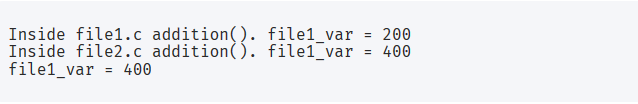Output extern c example

## strtok Fucntion in c with Program example for strtok

•  strtok function in c programming will help you to give the token of given input.
• This kind of function (strtok in c ) is very useful which reduce the length of c program.

# Let's see first : -

```#include `<string.h>`

char* strtok(char* str, const char* delim);```
• This function take an input str and also a delimiter character delim.
• Then strtok() in c split the input string into the tokens which is based on the delimited character.

# Return Value of strtok function in c

• This function return a single string.
• strtok() in c call this function continuously until we get NULL input sting.

# Example for strtok function in c

```#include `<stdio.h>`
#include `<string.h>`

int main() {
// Our input string
char input_string[] = "Hello from function in c!";

// Our output token list
char token_list;

// We call strtok(input, delim) to get our first token
// Notice the double quotes on delim! It is still a char* single character string!
char* token = strtok(input_string, " ");

int num_tokens = 0; // Index to token list. We will append to the list

while (token != NULL) {
// Keep getting tokens until we receive NULL from strtok()
strcpy(token_list[num_tokens], token); // Copy to token list
num_tokens++;
token = strtok(NULL, " "); // Get the next token. Notice that input=NULL now!
}

// Print the list of tokens
printf("Token List:\n");
for (int i=0; i < num_tokens; i++) {
printf("%s\n", token_list[i]);
}

return 0;
}```

We use the strtok(NULL, " ") function in c and use the loop for until the get NULL.

## Output strtok in c

```Token List:
Hello
from
Function
in
c```

## Example program for map() function in python

map() function in Python applies a given function to every item of an iterable and give a list of results.

## Syntax:-

`map(function, iterable, ...)`

# Parameter For map() function in python

map() function use two Parameter these are follow : -

•  function is a first Parameter map() function passes every item of iterable to the given function.
• iterables are second which is going to to be mapped.

Return value : - map() function in python return the list of result.

## Example of map() function in python

```def calculateSquare(n):
return n*n

numbers = (1, 2, 3, 4)
result = map(calculateSquare, numbers)
print(result)

# converting map object to set
numbersSquare = set(result)
print(numbersSquare)```

## OUTPUT : -map() function output

Well Python lambda Function commonly used with map () python function.